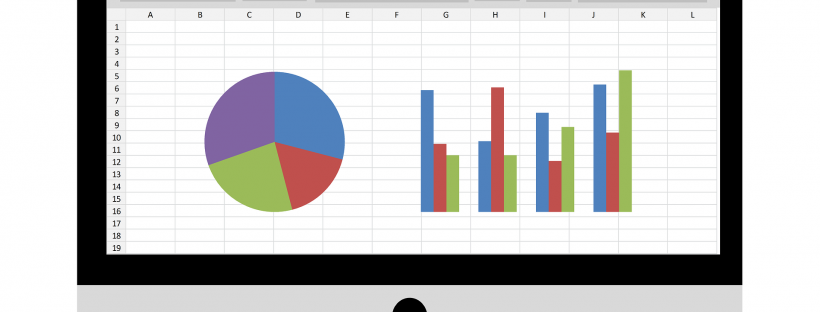# 5 Regression Techniques That Every Graduation Student Should Know

A student of statistical modeling needs to know and understand Regression analysis. This is used to find out the relationships which are there between variables. There are different kinds of techniques which can be used for analyzing and modeling several variables and this is also when the relationship needs to be focused on the independent variables and the dependent variables.

Regression analysis is also extensively used to forecast and predict. There are various techniques which are developed for carrying out a regression analysis. These are:

1. Linear Regression: This has a relationship established between the dependent variable Y and the independent variable X. There could be more than one independent variables involved. This is usually represented by Y=a+b*X+e in this a is the intercept, the slope of the line is b, the error term is e and the value of the target variable is then predicted.
2. Logistic Regression finds a probability of the event which is denoted by Success or failure. Logical regression is used when the dependent variable is in binary form – 0 or 1, true or false, yes or no. ln(odds)=ln(p/(1-p)). This is widely used to classify problems. In this linear relationships are not required between dependent and the independent variables. Various kinds of relationships can be handles.
3. Polynomial regression is used when the power of the independent variable exceeds 1. Y=a+b*xï 2. The best fit line is a curve and that fits the data points. It is not a straight line. Higher polynomials could end up with producing results which are weird on extrapolation.
4. Stepwise regression is where there are various independent variables. In this, the independent variables are selected by automatic means rather than human intervention. This maximizes the predictive power and that too with least amount of predictor variables. This handles higher dimensionality of the data which is there. In this, the predictors are added and removed as needed in each of the steps. The forward selection begins with the most significant predictor and the variable is added for each step. The backward elimination begins with predictors and the least significant variables are removed for each of the steps.
5. Ridge regression is used when there is multicollinearity of data. In this, the independent variables are highly correlated. Thus the OLS or least square estimates are unbiased and there are large variances and this deviates when it comes to the observed from the true value. Therefore, when a degree of bias is added to the estimates, the standard errors are reduced. In this, the assumption is the same as that of the least squares regression. However, here normality should not be assumed. Also, the value of the coefficients are shrunken but they do not reach zero.

These techniques are hard to grasp and to put into practice as well as the assignments do take time to complete when one does not have a firm grasp of these techniques in question and therefore most students opt for Statistic assignment help.

## 4 thoughts on “5 Regression Techniques That Every Graduation Student Should Know”

1. home family recipes says:Great article, totally what I was looking for.

2. mobile phones online says:Your style is very unique in comparison to other people I have read stuff from. I appreciate you for posting when you’ve got the opportunity, Guess I will just book mark this page.

3. web hosting says:You made certain nice points there. I did a search on the matter and found most people will go along with with your blog.

4. Justin says:Amazing Post